• ## matlab求定积分

千次阅读 2019-12-22 10:47:29
f在区间[a,b]上对t的定积分。 符号解：例如f=t^2*exp(-t);a=0,b=1 >> clear >> syms t%定义符号变量 >> f=t^2*exp(-t); >> int(f,t,0,1) ans = 2 - 5*exp(-1) 数值解：例如例如f=t^2*...
求f在区间[a,b]上对t的定积分。
符号解：例如f=t^2*exp(-t);a=0,b=1
>> clear
>> syms t%定义符号变量
>> f=t^2*exp(-t);
>> int(f,t,0,1)

ans =

2 - 5*exp(-1)
数值解：例如例如f=t^2*exp(-t^3);a=0,b=1
>> clear
>> t=0:0.0001:1;%中间的0.0001是步长
>> f=t.^2.*exp(-t.^3);%求节点处的函数值。这一句的"^"和"*"前面的"."表示对应元素乘方、相乘。因为t是向量，只有方阵才可以乘方，矩阵乘法也必须满足相应的维数关系
>> sum(f*0.0001)%节点处的函数值相当于矩形的高，步长相当于矩形的宽，乘积再求和就得到总面积的近似值。

ans =

0.2107

作者：TianSL
展开全文• 今天小编就为大家分享一篇python、Matlab求定积分的实现，具有很好的参考价值，希望对大家有所帮助。一起跟随小编过来看看吧
• matlab求定积分与不定积分 创建于2018-03-21 22:42 求定积分与不定积分是一件比较繁琐的事，但是我们可以借助matlab，下面与大家分享解决方法 材料/工具 matlab 不定积分 函数“xe^x”的不定积分 ...

matlab求定积分与不定积分
创建于2018-03-21 22:42
求定积分与不定积分是一件比较繁琐的事，但是我们可以借助matlab，下面与大家分享解决方法
材料/工具
matlab
求不定积分
求函数“xe^x”的不定积分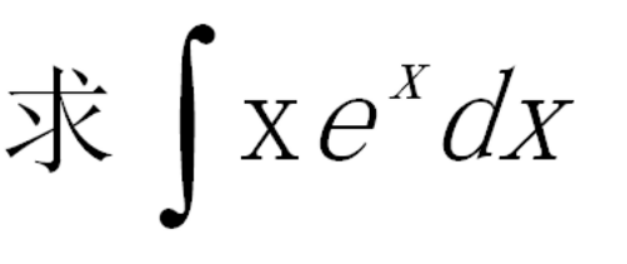要用到"int"命令，具体操作见下图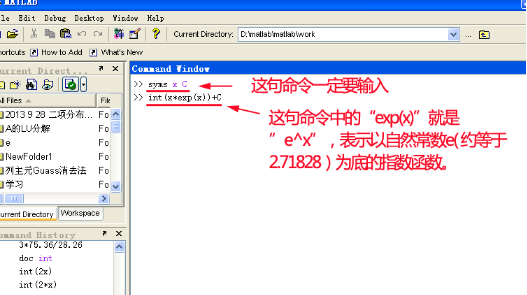函数“xe^x”的不定积分的结果如下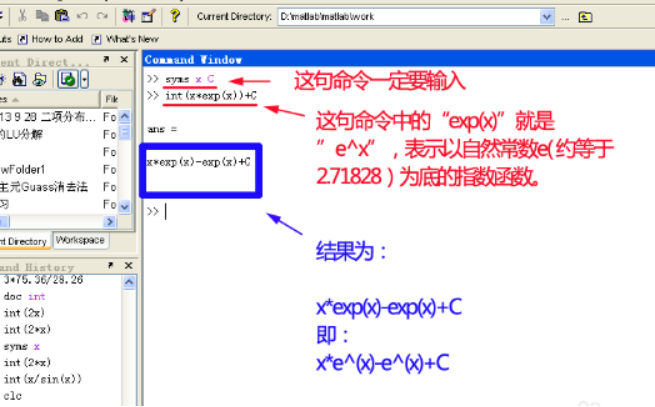求定积分
求函数"x^2*e^x"在（0到1）上的积分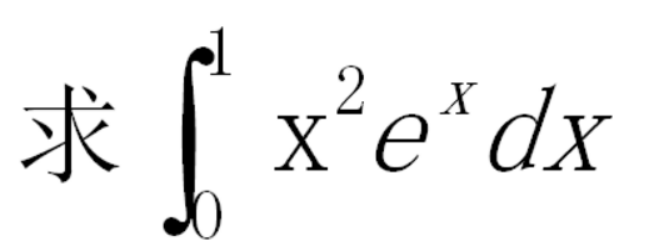具体操作见下图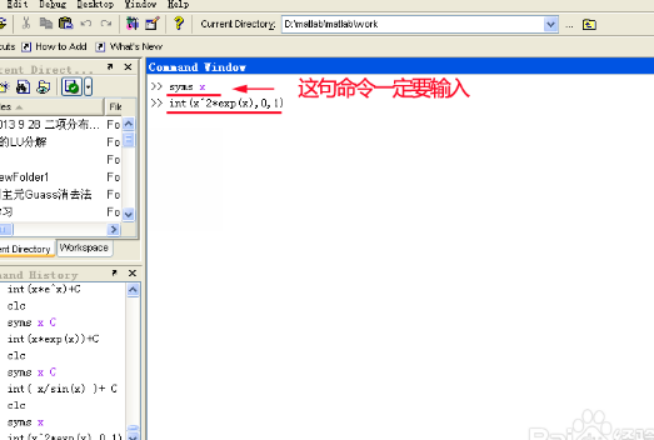函数“x^2*e^x” 在（0到1）的定积分的结果见下图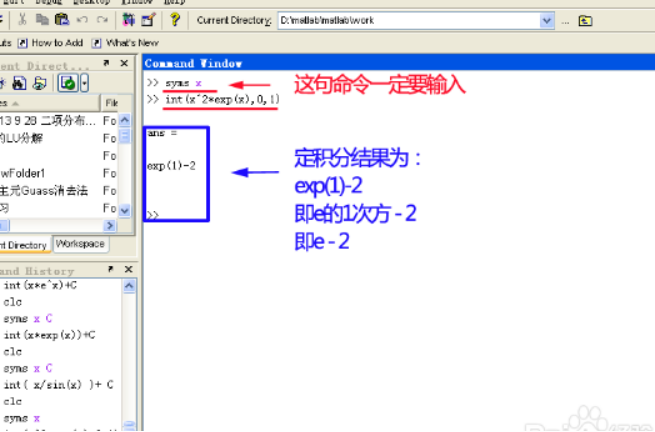转载于:https://www.cnblogs.com/henuliulei/p/9473505.html
展开全文• ## 用MATLAB求定积分

万次阅读 多人点赞 2017-12-21 10:57:20
一、符号积分 符号积分由函数int来实现。该函数的一般调用格式为： int(s)：没有指定积分...int(s,v,a,b)：求定积分运算。a,b分别表示定积分的下限和上限。该函数被积函数在区间[a,b]上的定积分。a和b可以是两个

一、符号积分 符号积分由函数int来实现。该函数的一般调用格式为： int(s)：没有指定积分变量和积分阶数时，系统按findsym函数指示的默认变量对被积函数或符号表达式s求不定积分； int(s,v)：以v为自变量，对被积函数或符号表达式s求不定积分； int(s,v,a,b)：求定积分运算。a,b分别表示定积分的下限和上限。该函数求被积函数在区间[a,b]上的定积分。a和b可以是两个具体的数，也可以是一个符号表达式，还可以是无穷(inf)。当函数f关于变量x在闭区间[a,b]上可积时，函数返回一个定积分结果。当a,b中有一个是inf时，函数返回一个广义积分。当a,b中有一个符号表达式时，函数返回一个符号函数。 例： 求函数x^2+y^2+z^2的三重积分。内积分上下限都是函数，对z积分下限是sqrt(x*y)，积分上限是x^2*y；对y积分下限是sqrt(x)，积分上限是x^2；对x的积分下限1，上限是2，求解如下： >>syms x y z  %定义符号变量 >>F2=int(int(int(x^2+y^2+z^2,z,sqrt(x*y),x^2*y),y,sqrt(x),x^2),x,1,2)  %注意定积分的书写格式 F2 = 1610027357/6563700-6072064/348075*2^(1/2)+14912/4641*2^(1/4)+64/225*2^(3/4)    %给出有理数解 >>VF2=vpa(F2)  %给出默认精度的数值解 VF2 = 224.92153573331143159790710032805   二、数值积分 1.数值积分基本原理 求解定积分的数值方法多种多样，如简单的梯形法、辛普生(Simpson)法、牛顿－柯特斯(Newton-Cotes)法等都是经常采用的方法。它们的基本思想都是将整个积分区间[a,b]分成n个子区间[xi,xi+1]，i=1,2,…,n，其中x1=a，xn+1=b。这样求定积分问题就分解为求和问题。 2.数值积分的实现方法 基于变步长辛普生法，MATLAB给出了quad函数来求定积分。该函数的调用格式为： [I,n]=quad('fname',a,b,tol,trace) 基于变步长、牛顿－柯特斯(Newton-Cotes)法，MATLAB给出了quadl函数来求定积分。该函数的调用格式为： [I,n]=quadl('fname',a,b,tol,trace) 其中fname是被积函数名。a和b分别是定积分的下限和上限。tol用来控制积分精度，缺省时取tol=0.001。trace控制是否展现积分过程，若取非0则展现积分过程，取0则不展现，缺省时取trace=0。返回参数I即定积分值，n为被积函数的调用次数。 例： 求函数'exp(-x*x)的定积分，积分下限为0，积分上限为1。 >>fun=inline('exp(-x.*x)','x');  %用内联函数定义被积函数fname >>Isim=quad(fun,0,1)  %辛普生法 Isim =   0.746824180726425 IL=quadl(fun,0,1)   %牛顿－柯特斯法 IL = 0.746824133988447   三、梯形法求向量积分 trapz(x,y)—梯形法沿列方向求函数Y关于自变量X的积分(向量形式，数值方法)。 >>d=0.001; >>x=0:d:1; >>S=d*trapz(exp(-x.^2)) S=  0.7468 或： >>format long g >>x=0:0.001:1;  %x向量，也可以是不等间距 >>y=exp(-x.^2);   %y向量，也可以不是由已知函数生成的向量 >>S=trapz(x,y);   %求向量积分 S =     0.746824071499185      附：int与quad区别 int的积分可以是定积分，也可以是不定积分（即有没有积分上下限都可以积）可以得到解析的解，比如你对x^2积分，得到的结果是1/3*x^3，这是通过解析的方法来解的。如果int(x^2,x,1,2)得到的结果是7/3   quad是数值积分，它只能是定积分（就是有积分上下限的积分），它是通过simpson数值积分来求得的（并不是通过解析的方法得到解析解，再将上下限代入，而是用小梯形的面积求和得到的）。如果f=inline('x.^2');quad(f,1,2)得到的结果是2.333333，这个数并不是7/3   int是符号解，无任何误差，唯一问题是计算速度；quad是数值解，有计算精度限制，优点是总是能有一定的速度，即总能在一定时间内给出一个一定精度的解。

展开全文• python求定积分 计算 from sympy import * x = symbols('x') print(integrate(sin(2*x)/(1+x**2), (x, 0, 3))) sympy库中integrate函数 integrate(f, (x, lower_bound, upper_bound)) # f-函数，x-变量，lower_...
python求定积分
计算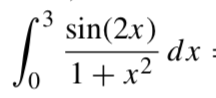from sympy import *
x = symbols('x')
print(integrate(sin(2*x)/(1+x**2), (x, 0, 3)))

sympy库中integrate函数
integrate(f, (x, lower_bound, upper_bound))
# f-函数，x-变量，lower_bound-下限，upper_bound-上限

但是发现求不出来，如果是sin(2*x)就可以，为什么？

matlab求定积分
syms x
f = sin(2*x)/(1+x^2)
int(f, x, 0, 3)

或者：
syms x
f = @(x) sin(2*x)/(1+x^2) #句柄
integral(f, 0, 3)

同样得到的是要么是化简之后的结果，要么报错，说明这个函数不可能得到理论解。
展开全文• 一个积分，能出解析解固然好，但是不出解析解，一个数值解基本能够满足实际的需求...下面以椭圆积分为例，我来说一说，matlab如何求定积分。 clc clear syms theta; a=5; b=3; c=sqrt(a^2-b^2); e=c/a;h p=abs(a算法
• 一、符号积分符号积分由函数int来实现。该函数的一般调用格式为：int(s)：...int(s,v,a,b)：求定积分运算。a,b分别表示定积分的下限和上限。该函数被积函数在区间[a,b]上的定积分。a和b可以是两个具体的数，也可以...
• 这个函数里面存在贝塞尔函数 function f=abs_R(lamda) x=0:lamda/100:lamda; y=1./tan(-1.*besselj(1,x)./bessely(1,x))./(x.*sqrt(lamda.*lamda-x.*x)); % y=@(x)1.0./tan(-1.*besselj(1,x)./bessely(1,x))./(x.*...有问必答
• 假定用矩形或梯形拟合吧% function yanshi(fname,a,b,n)% %积...给你一个例子。x=-1:0.05:1的积分区间划成步长为0.05的41个等分y=exp(-x...dx=0.1;x=0:dx:4;y=x.*sin(x);s=cumtrapz(y)*dx; %梯形法...quadgk(@(x)x...
• 一、符号积分符号积分由函数int来实现。该函数的一般调用格式为：int(s)：...int(s,v,a,b)：求定积分运算。a,b分别表示定积分的下限和上限。该函数被积函数在区间[a,b]上的定积分。a和b可以是两个具体的数，也可以...
• 原创Matlab计算定积分的操作内容讲解编辑:小鑫 来源:PC下载网时间...打开Matlab，找到Matlab的命令窗口，点击进入，如图，当然也可以编辑脚本然后通过运行脚本来求定积分。需要在命令行输入syms x， sym的作用是将字...
• 实验4 利用matlab计算不定积分 一运算命令 二例题讲解 实验5 利用matlab计算不定积分 一运算命令 1.Matlab求解定积分 2.Matlab求解广义积分 二例题讲解 一本章知识点 说明该命令得出的结果F(x是积分原函数实际的不定...
• 不定积分与定积分 %%计算不定积分 clc; clear; syms x; % 定义一个符号 x。 f = 'x'; % 定义一个原函数 F = int(f, x); % 计算不定积分 disp(F); % 显示原函数 %%计算定积分 clc; clear; syms x; % 定义一个符号 ...
• 使用Matlab求解定积分/不定积分 ...用MATLAB求定积分 https://blog.csdn.net/u010999396/article/details/78861212 MATLAB解决微积分问题 https://blog.csdn.net/karry_zzj/articl...
• 如何用matlab计算定积分如何用matlab计算定积分如何用matlab计算定积分
• ## matlab int 求定积分

万次阅读 2018-06-28 11:21:29
int(s,v,a,b)：求定积分运算。a,b分别表示定积分的下限和上限。该函数被积函数在区间[a,b]上的定积分。a和b可以是两个具体的数，也可以是一个符号表达式，还可以是无穷(inf)。当函数f关于变量x在闭区间[a,b]上可积...
• matlab定积分的近似计算.ppt MATLAB数学建模与仿真 定积分的近似计算 2 定积分计算的基本公式是牛顿 莱布尼兹公式 但当被积函数的原函数不知道时 如何计算 这时就需要利用近似计算 特别是在许多实际应用中 被积函数......

# matlab求定积分matlab 订阅